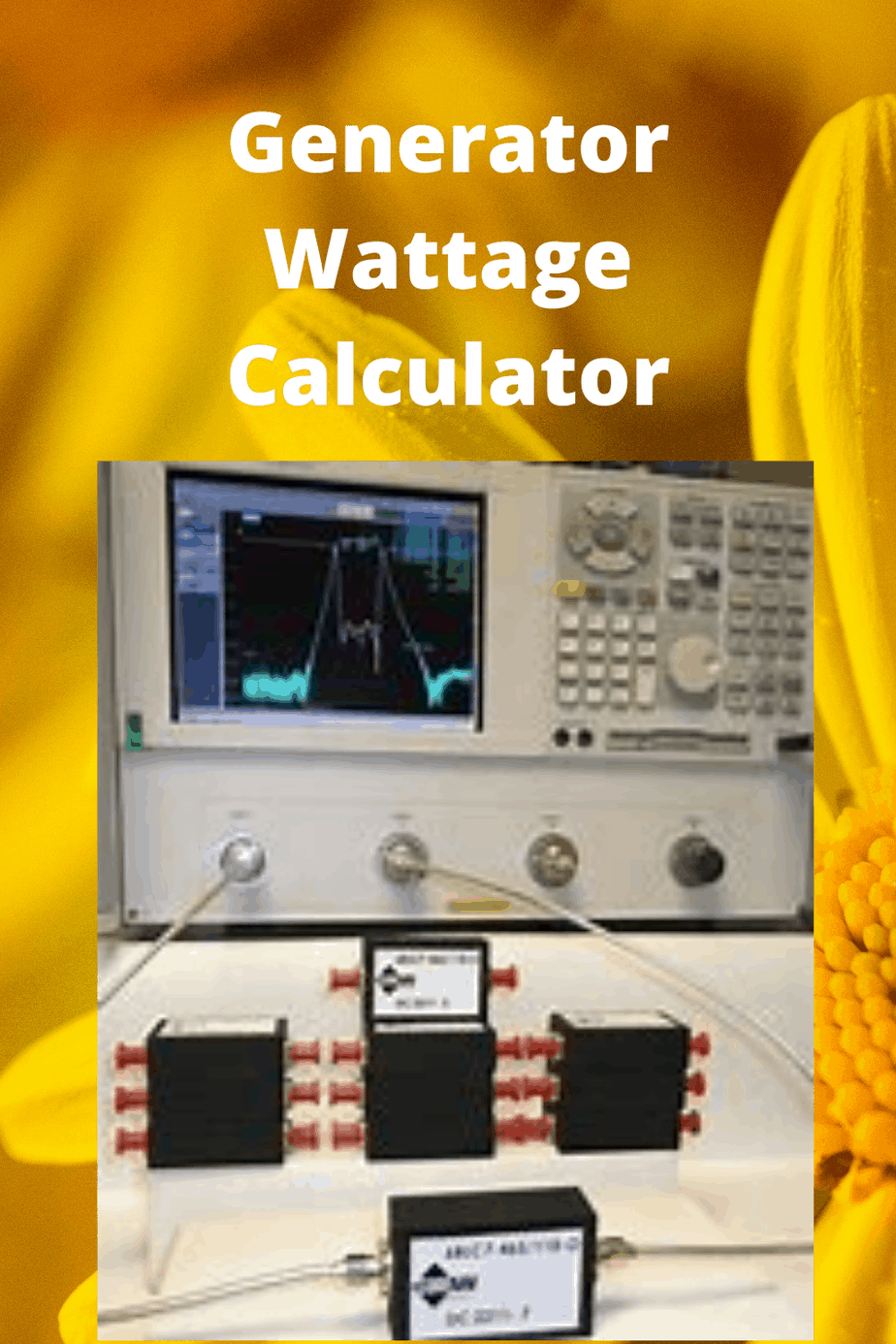# Generator Wattage Calculator OnlineGenerator Calculator

## Wattage Calculator

Contents

#### What do you want to power?

Quantity

Item Qty Starting Wattage Running Wattage
Total

#### Recommendations :

Recommended wattage:
Maximum wattage:
best generator for home on AmazonDisclaimer: Every time, it comes to electric and power calculations, it is a good idea to get advice from an expert for your specific situation.

The calculations on this web page are for general estimations only.

What is a generator wattage calculator?

Generator wattage calculator is a calculator that will help you to determine the amount of power that you need for your house, your work or your recreation area.

How to use it?

1. First, you select one among these three (Property, Amusement, Labor)
2. Next, you checkmark all the devices that you are using and select the number of each.
3. Lastly, you hit calculate, it will display the result on the screen.

### Wattage

We hear the term Watt a lot when talking about power, or electricity.

Whether it’s in reference to the light bulb (e.g the 60 Watt bulb) or in a broader way (e.g: the term Wattage) we are often left wondering what exactly is a Watt?

Well, A Watt is a basic unit for measuring power.

The Watt was named for Scottish inventor James Watt, whose inventions contributed greatly to the industrial revolution, in 1908.

A Watt is roughly equal to one Joule.

### This begs the question of what is a Joule?

A Joule is the amount of energy exerted when one Newton of force is applied over the displacement of one meter.

So, what is a Newton? A Newton is a unit of force. So, basically a Watt is the corresponding amount of Power to a Joule’s energy and Newton’s force.

Although they produce the same amount of energy they do so differently.

Basically a Watt is how we measure power, whether it’s the brightness of a light bulb, the speed at which a fan’s blades turn, how strong an individual is, or the fuel efficiency in our cars Watts is power. Watts is how we burn energy and how we use that energy.

How do you calculate Wattage? to determine the amount of Watts in a power source you need to know the AMPS and the Volts.

The Ampere ( AMPS), or the amount of electricity used is multiplied by the Volts, the force or how strong the electricity and this equals your Wattage.

It’s a simple multiplication problem. Watts = AMPs x Volts (W= A x V)This formula can also be written as Power=AMPs x Volts (P= A x V).

P in this instance standing for power, because Watts is the measure of power. Power and Watts are interchangeable for this equation.

### Generator Sizing Calculator

This calculator is a device or tool that allows you to calculate the size of a generator to determine its power through an electric charge.

It is necessary to know the parameters around it to use this tool in the right way. It is essential to know the sequence and boot method of the motors or generators to be calculated.

Conversions

Usually, this device works with several types of conversions:

From kVA (kilovolt ampere) to kW (kilowatt).

From Kw to HP (horsepower).

Ampere (when the value of kVA is known).

Generator size

It will depend on the use of all its applications. The overvoltage power of each motor must be known.

It will only be necessary at the beginning. The rest will start thanks to the switching system automatically.

The generator sizing calculator is essential because it allows us to know the size and power that an engine can withstand.

That can be very useful in some emergency power outage. With the tool, you can determine the number of watts needed to power a place.

Related

Kilowatts to Kilovolt Amps Calculator

Kilovolt Amps to Kilowatts Calculator

Watts to KVA Calculator

ton to kw